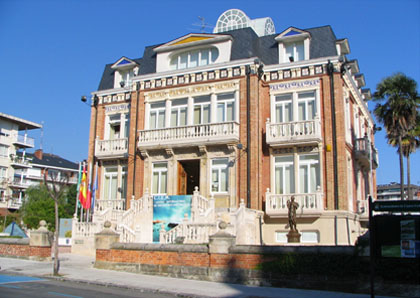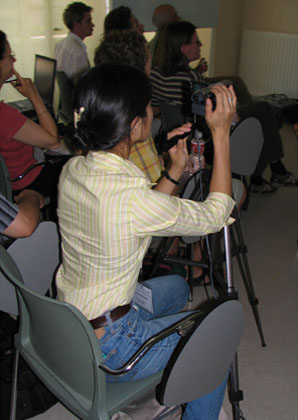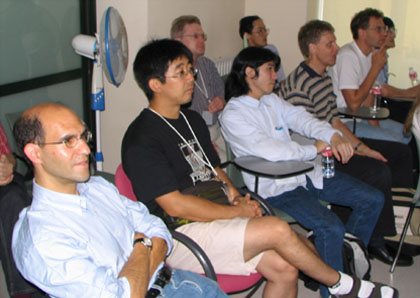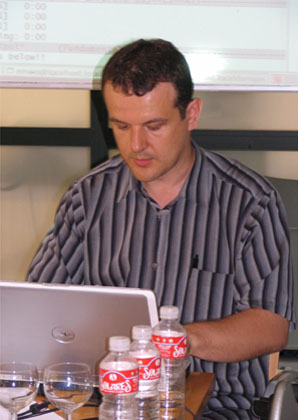# Proof assistant demos at ICMS 2006## Exercise

Problem [B2 from IMO 1972]
f and g are real-valued functions defined on the real line. For all x and y,

f(x + y) + f(x - y) = 2 f(x) g(y).

f is not identically zero and |f(x)| ≤ 1 for all x. Prove that |g(x)| ≤ 1 for all x.

Solution
Let k be the least upper bound for |f(x)|. Suppose |g(y)| > 1. Then

2k ≥ |f(x + y)| + |f(x - y)| ≥ |f(x + y) + f(x - y)| = 2 |g(y)||f(x)|,

so |f(x)| ≤ k/|g(y)|. In other words, k/|g(y)| is an upper bound for |f(x)| which is less than k. Contradiction.

## Demos1. ### Isabelle, try-out by Jeremy Avigad2. ### HOL Light, demo by John HarrisonVideo of the demo

3. ### Mizar, demo by Artur KornilowiczVideo of the demo

4. ### ProofPower, demo by Rob ArthanVideo of the demo

• Polished version with both the demo proof and Hales' proof: pdf doc (doc does not mean "Word" here, but "ProofPower") (formalization times: about 77 minutes and about 35 minutes; de Bruijn factors: about 2.4 and about 2.0)
5. ### Coq, demo by Laurent ThéryVideo of the demo

(last modification 2006-09-21)